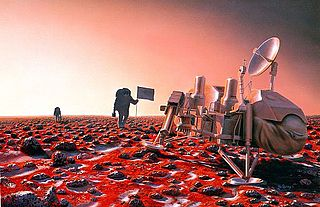# An astronaut on planet XAn astronaut lands on planet X which has $\frac34$ the mass and $\frac34$ the radius of Earth. On Earth the astronaut weighed $900\ N$. How much does he weigh on planet X?

×